# N8PPQ

## My Personal Site

The Pattern for a
Spiked Flail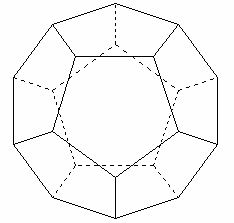This dodecahedron provides the pattern I want for the spikes on a flail. The sphere is circumscribed. One spike corresponds to each node, where three edges meet. Note that a great circle will transverse above the altitudes of four pentagons and two edges. The job of locating these nodes on a ball of known diameter involves four equations and four variables.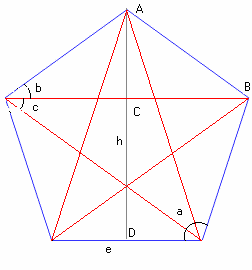This is a crummy drawing. I used MS Paint because it's convenient. We need to relate the edge e to altitude h.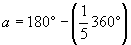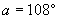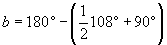,
Since the angles of a triangle (ABC) add to 180º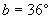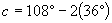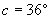,
which may or may not have been obvious.

Now, to get the base e in terms of h.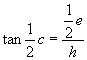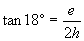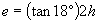Equation 1Equation 1:Now to relate the edge and altitude of the pentagons to the radius of the ball.  We will need to relate the central angles u and w.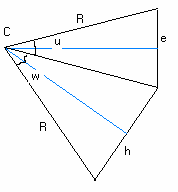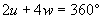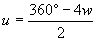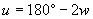Equation 2

Now using trigonometry of right triangles we see that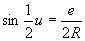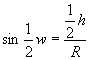Equation 3

and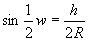Equation 4

So, we have four equations relating the four variables e, h, u, w
We'll use substitution to put Equations 3 and 4 in terms of e and w and solve both for e.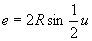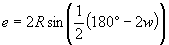from Eq. 2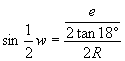from Eq. 1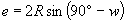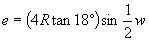(we cannot avoid a half angle or double angle calculation)

Now, setting these two expressions for e equal we have an equation in one variable. This we (should be able to) solve.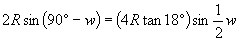.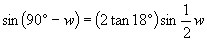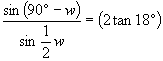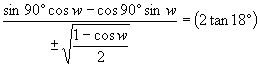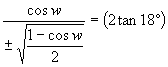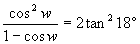I was surprised to see the R's cancel, but of course       they should. The central angles are not related to the radius.   Now we invoke the identies: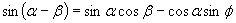and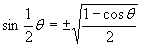.   Now sin 90º = 1, of course, and cos 90º = 0.  Now square both sides to get rid of the radical.   We'll have to check our results for extraneous solutions.   I looked at this for a while. I expected the Pythagorean identity to help, i.e.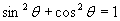, hence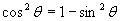,   which factors thus: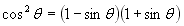, neither of which cancels the denominator.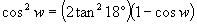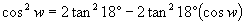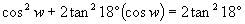So, I rearranged the terms to look like a quadratic equation in cos(w).    Now I'll use the "completing the squares" technique.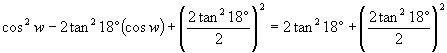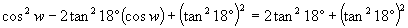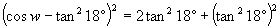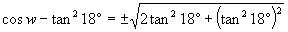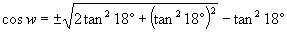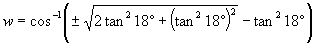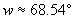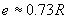By taking the square root of both sides.   Time to find a calculator.    Not bad!    Now we'll plug this in to the equationto find our edge e. We're done!   For the 3" ball, R = 1.5" and   fini!

Now, we know the length of the pentagon edges. My thought was to layout a rectangular piece of sheet steel with five divisions this size, then to bend it into a sort of cookie-cutter. I considered laying this on the ball, in order to locate the nodes. I planned to undercut the sides of the cookie-cutter in order to bring its corners close to the ball's surface.

Click on the link below to see the real thing.